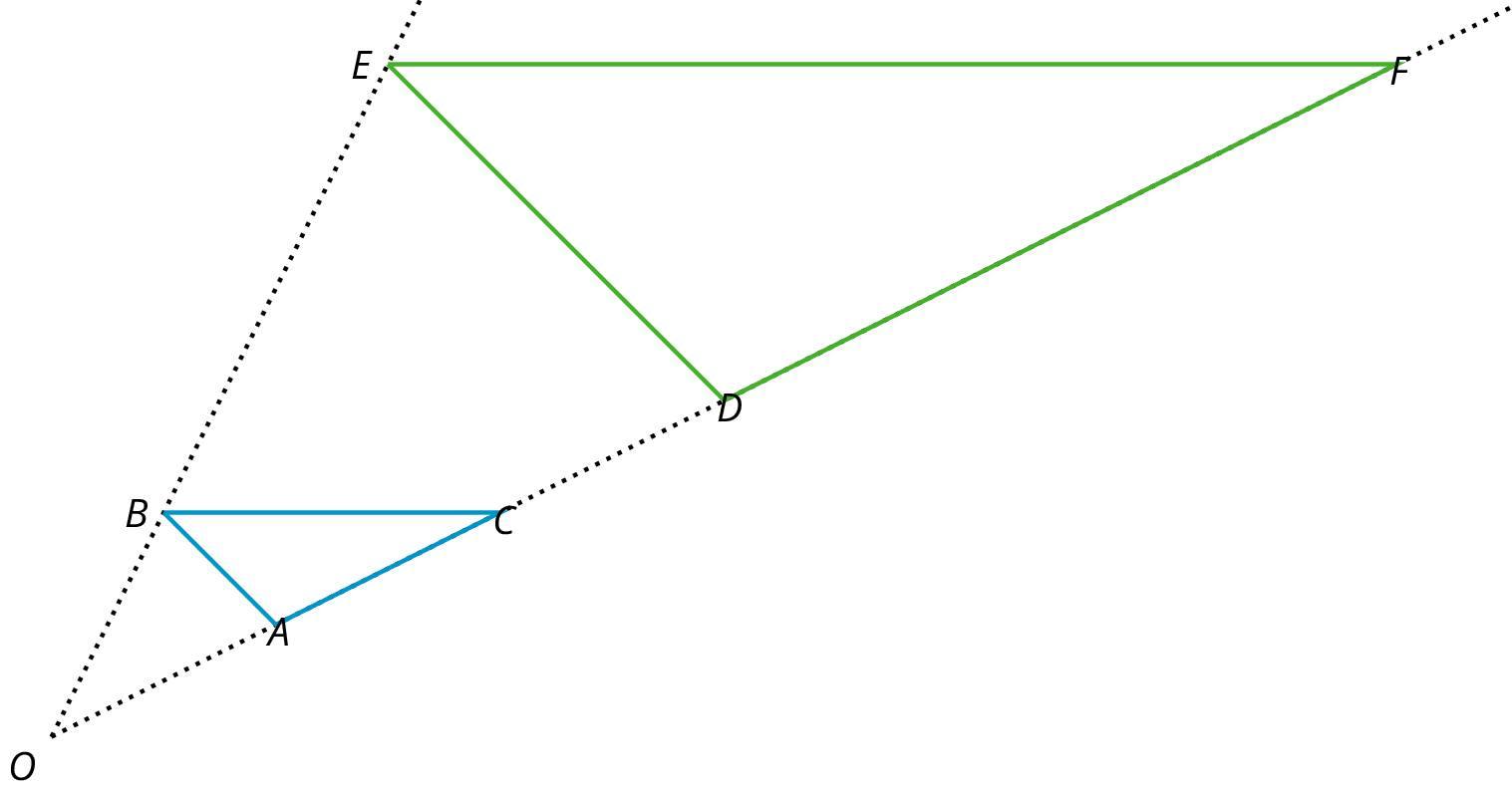# Lesson 1

## Introduction to Dilations

Dilation and Center (of Dilation) - A dilation with center O and positive scale factor r takes a point P along the line OP to another point whose distance is times further away from O than P is. If r<1 then the new point is really closer to O, not further away.  The triangle DEFis a dilation of the triangle ABC with center O and with scale factor 3. So D is 3 times further away from O than A is, Eis 3 times further away from O than Bis, and F is 3 times further away from O than C is.## Dilate the figure by 2, and the dilation point as A. Then dilate it by 1/2 and the point as A. What do you notice?

Dilate the figure by 2, and the dilation point as A. Then dilate it by 1/2 and the point as A. What do you notice?